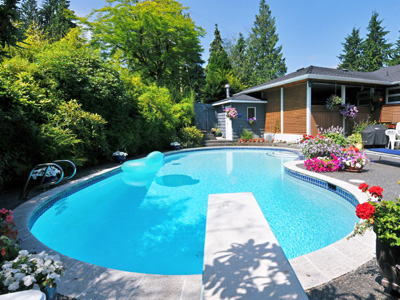Liters are the best units to measure the volume of water in a swimming pool.

# Measures 3

This Math quiz is called 'Measures 3' and it has been written by teachers to help you if you are studying the subject at middle school. Playing educational quizzes is a fabulous way to learn if you are in the 6th, 7th or 8th grade - aged 11 to 14.

It costs only \$12.50 per month to play this quiz and over 3,500 others that help you with your school work. You can subscribe on the page at Join Us

In middle school Math as children advance, their knowledge of measures will increase. By now they should be very familiar with the metric measures (millimeters, centimeters, meters, kilometers, grams, kilograms, milliliters and liters) and should be comfortable combining measures to write measurements (eg. 1.5kg = 1kg 500g) and rounding smaller measurements up or down into larger ones (eg. 740m to 1km).

Grams and kilograms are used to measure the mass or weight of things. We can write the same weight in kg (1.6kg), in g (1,600g) or by combining the two (1kg 600g). Rounding fractions of larger measures to the nearest whole number is another way of writing measurements. 1.6kg would be rounded up to 2kg. Metric and imperial measurements are used all the time in day to day life. To know your grams from your ounces and your meters from your miles will stand you in good stead, so keep practicing these quizzes on measures.

How are you at combining m with km or rounding ml to the nearest l? Do you know your metric measures from your imperial ones? See how well you do in this quiz on measures.

1.
What is three quarters of 1 meter in cm?
0.75cm
75cm
750cm
7,500cm
Three quarters of 100 is 75
2.
What is 4,321g to the nearest kg?
4kg
5kg
43kg
433kg
As there are 1,000 g in a kg, this is the same as rounding to the nearest thousand
3.
Roughly how many liters in 1 gallon?
4 liters
5 liters
8 liters
10 liters
A gallon is slightly less than 5 liters
4.
What is 156cm to the nearest meter?
1m
2m
15m
16m
As there are 100 cm in a m, this is the same as rounding to the nearest hundred
5.
Which unit is best to measure the volume of water in a swimming pool?
kg
ml
cm
liter
There may be 1,000s of liters of water in a swimming pool
6.
Which of these is best measured in cm?
The length of a pencil
The length of the school playground
The weight of a bag of flour
The height of a multi-storey parking lot
The length of the school playground and the height of a multi-storey parking lot would both best be measured in meters. The weight of a bag of flour should be measured in kilograms
7.
What is 6.45kg expressed in grams?
0.645g
64.5g
645g
6,450g
To convert kilograms to grams, times by 1,000
8.
How many grams are equivalent to one hundredth of a kg?
0.1g
1g
1.1g
10g
There are 1,000g in a kg so 1,000 ÷ 100 = 10
9.
Which of these statements is true?
1 mile > 2 km
1 mile < 1.5 km
1 mile > 1.5 km
1 mile = 1.5 km
1 mile is more than 1.5 km but less than 2 km
10.
How do we write 4,520ml in liters?
0.452 l
4.52 l
45.2 l
452 l
To convert milliliters to liters, divide by 1,000
Author:  Amanda Swift